I've always felt that this subject is presented badly. Since this isn't likely to be much of an exception, do let me know if you have any questions about (or tips on how to present) the material!

Work in progress...

## Motivation

Like much mathematical notation, differential forms exist because they are the natural way to consolidate what looks like lots of vaguely connected but visibly different ideas into a single compact framework which enables the creation of a language and notation in which lots of concepts look neater.

The prototypical examples of the simplification offered by differential forms are probably Stokes's theorem (for mathematicians) and Maxwell's equations (for physicists).

Note: Throughout this article, I use an italic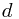for the 'exterior derivative' and related notation. This is purely because it's easier to typeset in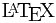. There is a controversy over whether it should be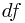or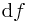(or something else with different spacing again), mainly because technically it is an operator, and so should be upright, but in practice nobody can be bothered.

Other note: I use the summation convention so that repeated indices indicate summation.

### Stokes's theorem

There are a few theorems in (multivariate) calculus which have a very similar flavour, but which do not have an obvious generalization. The theme is this: "integration of a derivative over a volume is the same as integration of the original function over the boundary of the volume". The two most commonly referred to versions of this are the 1D and 3D versions of the more general "divergence theorem":

• Fundamental theorem of calculus -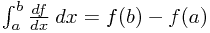• Divergence theorem -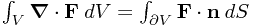where the volume V is bounded by the surface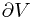with unit outward normal n.

Can you see the similarity? If we make sure we think of the interval [a,b] in the 1D real line as having a 'surface' consisting of the two points a and b, and integration over these points as being a sum, then these are actually exactly the same statement! Note that the outward normal at a is the vector (-1). Since everything is 1D, the rest of the expression simplifies a lot.

So far, so good. But then comes along Stokes's Theorem. This one works for surfaces in 3D...

• Stokes's Theorem (curl special case) -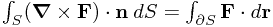where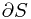is the curve around the border of the surface.

... oh dear. This really doesn't fit into the framework. Maybe it's an unrelated result? It turns out that this isn't the case. In the language we'll introduce below, we'll write both the divergence theorem and the above case of Stokes's theorem in one simplified form: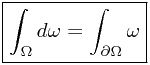All will be revealed!

### Maxwell's equations

I'll leave you to look up the various ways of writing Maxwell's equations, but by far the neatest, as we'll see below, is this: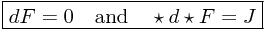(And yes, if you haven't seen it before, the Hodge star is a real thing.)

### Conceptual motivations

Finally, the underlying mathematical reasons why we might want to talk about things in terms of differential forms come in the form two related ideas, to my mind:

• Coordinate-free notions - Lots of statements - especially physical laws - are really properties of the world we live in, and not how we choose to look at it. So statements like Maxwell's equations ought to have a form which doesn't depend on what basis we're in - in fact, it should be possible to write them down without ever mentioning a basis. The usual notation doesn't do this very well; in particular, the Hodge star used in the above formulation of Maxwell's equation makes much more sense in this formalism than in the usual coordinate expressions.
• What a vector is - Our starting point below will be trying to pin down exactly what we mean by a "vector" when we're on a general manifold. The key idea is this - imagine you are on a sphere, and you want to point in a direction. Obviously, if you're human, you tend to point "in the tangent space" - that is, you point in a direction locally parallel to the ground. But this means technically pointing in a direction off the surface of the Earth. All well and good here, but if you're talking about the universe as a manifold (which it sure seems to be) then we don't really want to be pointing out of the universe... So we want an idea of a vector (field) which is entirely native to the world (manifold) we're living in.

## Intuitive Development

As suggested above, let's start with the notion of a vector. When we're in nice flat Euclidean space, the usual idea of an arrow connecting two points serves us well. But imagine you're at a point P on the surface of a sphere, a 2D world. Then what do you mean?

The usual approach involves considering the tangent space at that point; that is, we add the structure of a locally Euclidean (say) 2D plane lying on the sphere at P. But how do vectors in this plane correspond to ideas like velocity around the sphere - that is, to lines drawn on the sphere? Intuitively, we pick the vector in the tangent space which is to first order the same as the line in the embedded space - the tangent vector is tangent to the line in the manifold (the sphere).

But these notions all rely explicitly on the embedding into the higher-dimensional space, which - whilst possible - is not necessarily very natural, or even unique. So if we didn't know about the 3D world outside our sphere, how would we get a basis for the vectors here at point P? Given that we've seen the natural correspondence between lines on the sphere and vectors is driven by agreement to first order, perhaps we should try thinking about differentiation along these lines (literally).

Suppose we choose some nice local coordinates for the manifold M (which, recall, is the point of a manifold, although we don't actually have to pick locally Euclidean ones at all), say x1, ..., xn. Try to keep track of what sort of object everything is - the coordinates are functions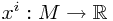. Now if we had an embedding, these coordinates (think of θ and φ on the unit sphere, for example) could be supplemented with extra ones (think of the coordinate r pointing away from the sphere) so that the original coordinates parametrized the surface, and the other ones were all orthogonal to it. But we don't worry about these extra coordinates; we just note that changing one of the original xi a little bit corresponds to wandering along the surface to first order, in the direction which would be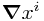in the embedding, but which for us will shortly define the vector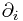. These will form a basis of the tangent space at the point P.

But if we're living entirely inside this surface, what can we look at changes in? We have to have functions defined on the manifold. We already have a pretty useful bunch - the coordinate functions xi. But we don't want to be tied to specific coordinates, so let's ask this question:

Given a tangent vector v at a point P, and a function f on M, what can we get out?

Pretty much the only possible answer to this question is: the change in f if we go in the direction of v!

Let's formalize this in a way that doesn't think about the embedding. Suppose you have a (C) function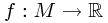. Then any derivation V at a point P - a first order differential operator, as we'll define in a moment - corresponds to an element of the tangent space via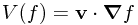at that point.

A derivation is basically what you expect, leading to the following definition of a vector field in terms of linearity and also the Leibniz property, which is what ensures it behaves like differentiation:

A vector field V on a manifold M is a function (or operator) from C(M) to C(M) such that
1.for all real α, β and f, g in C(M).
2.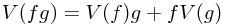for all f, g in C(M)

There we go! We have a notion of a vector field which is completely divorced from the usual notions in Euclidean space. A tangent vector is defined similarly, but as a map at a single point.

(And just for clarity: the C(M) functions here have no particular significance in general - we just want to be able to have something to differentiate.)

The wonderful thing about this is that it is completely basis-independent - however, it might be useful to be able to think about what sort of basis we might have for these operators.

This is easy enough to do - returning to our coordinate functions, we note that the vector fields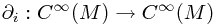which compute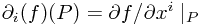form a natural basis. Be careful here - they are a basis in the sense that any vector field V can be written as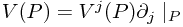where the components Vj are functions from the manifold M. That is, at each point P, the operators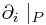form a basis for the tangent space.

We completely identify vectors in the tangent space with the differential operator giving the directional derivative along this vector.

### What next?

Now we have a concept of a vector field defined purely in terms of the manifold M. We've found ourselves thinking in terms of directional derivatives a lot. Since directional derivatives in Euclidean space are usually thought of as 'bits of the gradient' - i.e.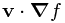for some vector v and function f - it's natural to ask whether the gradient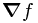can be given a concrete meaning in this framework.

The first incredibly important point is that gradients are not vectors in general manifolds. This can be a source of great confusion at first, but it's not too surprising if you're into relativity and index gymnastics. The simplest objection to thinking of gradients as vectors in this context is that they have the wrong sort of index: a (contravariant) vector has an upper index for its components, vj, whilst the gradient has a lower index: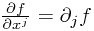. This matters because they transform differently.

The simplest way of seeing this is to remember that we know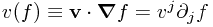is coordinate-independent; but if we, say, rescale our first coordinate by doubling its size, then the derivative gets half as big, so the vector component vj must double.

Now since we want to think in our coordinate-independent way, let's look at the expression v(f). If we want a notion of the gradient of f, it's now natural to express it as something which, given some vector v, spits out the rate of change along that direction.

The gradient is a covector or dual vector; it can be viewed as a map from the space of vectors to the real numbers. The formal definition uses a new piece of notation:

Letbe a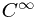function. The differential or 1-form of the function is df, the linear map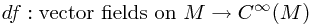defined by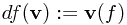Let's just rephrase that in words: df is the analogue of the gradient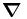for a general manifold - and when given a vector, it returns the component of the gradient along that vector. Note that this is indeed a linear map. Recall that you can view the Euclidean gradient in this way too: it is a row vector, which can be multiplied by a column vector using matrix multiplication to give a real number, the directional derivative. This is precisely analogous.

We denote the space of all 1-forms on a manifold M by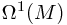, and for completeness we define the exterior derivative to be the d in the above expression:

The exterior derivative is the operator (linear map)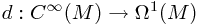defined by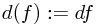It inherits linearity and the Leibniz property from the definition of vector fields; e.g.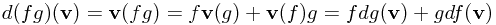so that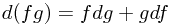### The 'small change' interpretation

Of course, now we've introduced some notation which overlaps with the conventional notation of df as a small change in f, or a differential. Does this make sense? Yes - because if we make any small change in our coordinates, so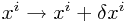, then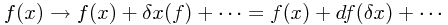. Hence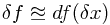. Then since df are all linear maps, an equality involving these corresponds to an equality involving the first order changes in the corresponding functions.

Essentially, we're just saying that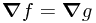implies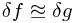to first order.

What about differentiation as 'dividing by differentials'?

This is not really a very mysterious process; we can just divide through the above equations by the small change in x, δx, and take the limit as this goes to zero. The dx term is, however, not really a differential here.

### Some examples of differentials

• Thermodynamics: You might be familiar with expressions like conservation of energy - the first law of thermodynamics - which is often written in the form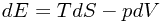which we would usually interpret as giving a small expression for small changes in the energy. In this framework, what does this mean?

The most important question is simply "What is the manifold M?" The answer is that it is the collection of macroscopic states - that is, values of E, T, S, p, V, ... - connected to the initial one by physical processes. For example, if entropy S and volume V allow us to determine p, T and E unambiguously given evolution from an initial state obeying all conservation laws, then the manifold is 2-dimensional, and could be viewed as a 2D surface forming the graph of p = p(S,V), T = T(S,V) and E = E(S,V) in a 5 dimensional space.

Now in this point of view, the first law of thermodynamics tells you about how the derivative of the graph 'height' E changes to first order as you tweak S and V.

Of course, if you then perform a new process violating this statement - because energy is exchanged with the environment - then the physics is no longer constrained to the manifold, and so the above formula no longer applies.

• Relativity: The other main example of the use of differentials is in the metric in general (or occasionally special) relativity, where we typically write things like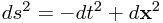which is the Minkowski (flat space) metric. This is generally interpreted as meaning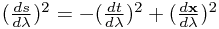but what meaning can we ascribe to it in the language of differential forms?

Firstly, the manifold - it is most natural to use the coordinates t, x to describe a 4D manifold, upon which we have some parametrized spacelike curve with some arbitrary starting point O. We can locally define a function s = s(P) giving the proper relativistic distance to other points P on the curve from the origin O. (Why only locally? Because in general, if the curve is e.g. circular, then one can arrive back at the starting point with a non-zero value for s!) Note that s is not defined on the whole manifold and as such does not have a true 1-form ds!

Morally, s should be thought of a parameter along a curve, not a function of the manifold. This is what makes it impossible to think of "ds" as a 1-form on the manifold; it has meaning only on a particular curve.

Now let's think about these squared terms in terms of Euclidean gradients. Clearly, to give the above interpretation, what we want to have is an equality between terms like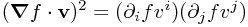. We want to write this so that the v terms drop out, so it is purely an equality involving the gradients/differentials. But it is easy to see in the index notation version that this works if we think of df2 as a quadratic form in the vj - that is, it's a linear function of two arguments which are here always the same. Another way of saying this is that df2 is the matrix with elements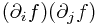; a caveat is that we only ever see the symmetric part if we always sandwich it between two vs.

What about mixed terms, like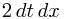? These are also fine, though taking the symmetric part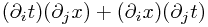is natural.

Then in general, if we have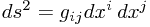, we obtain an equation for the equality of two matrices - or more properly tensors: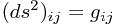and where we assume gij was chosen to be symmetric. We can view the metric g as a bilinear, symmetric map from the space of tangent vectors at each point to the real numbers, giving the first-order estimate of the squared proper distance along a tangent vector if it is given as both arguments. (Assuming the vector is spacelike.)

Again, we stress that "ds" is not a differential; clearly, a general metric tensor cannot be written as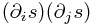anyway, as this is a very special type of tensor. Instead, it can be used to calculate the rate of change of s along a specific curve from a specific origin via taking the inner (matrix) product with the tangent vector on the left and right.

The formal notion of 'multiplication' used in writing terms like dt dx here is the (symmetrized) outer product or tensor product. We might formally write this: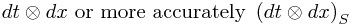The 'gradient-like' version of this would note that the matrix product of a column vector with a row vector is a matrix (as opposed to the product of a row vector with a column vector, which is a scalar). We're essentially considering matrices like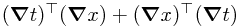or in coordinates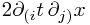where brackets (...) denote symmetrization in the form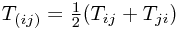.

For the general treatment of metric tensors, see the relevant Wikipedia article.

### Exact differentials

One last point worth mentioning is that not all 1-forms arise as differentials of functions - for example, on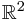, the 1-form y dx cannot be expressed as d(f(x, y)), the exterior derivative of a 0-form. (Note that smooth functions on the manifold are also called 0-forms.)

Why? Let's expand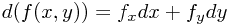. So if y dx is of this form, then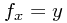and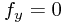. But partial derivatives commute, so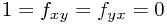which is a contradiction! We say y dx is not exact.

We will encounter below the concept of a closed differential, which is related in a non-obvious way.

You might like to show - using the simple version of Stokes's theorem above - that the commuting partial derivatives condition above is necessary and sufficient for a differential to be exact when the manifold M is. How does this relate to the form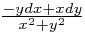and the angle θ to the x-axis?

## Integration and Wedge Products

Before we go any further, it would be good to understand how differential forms show up in line integrals.

This is easy enough to understand; if we have a parametrized curve C with parameter s (cf. the relativity example above), then the integral of a differential form ω along the curve is simplywhere v is the tangent vector along C (the rates of change of coordinates, say) and the integral over s is simply an integral over a real parameter.

If ω is exact, though, so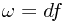, then the fundamental theorem of calculus and the above definition tell us that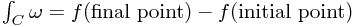which is as we would hope.

...

...